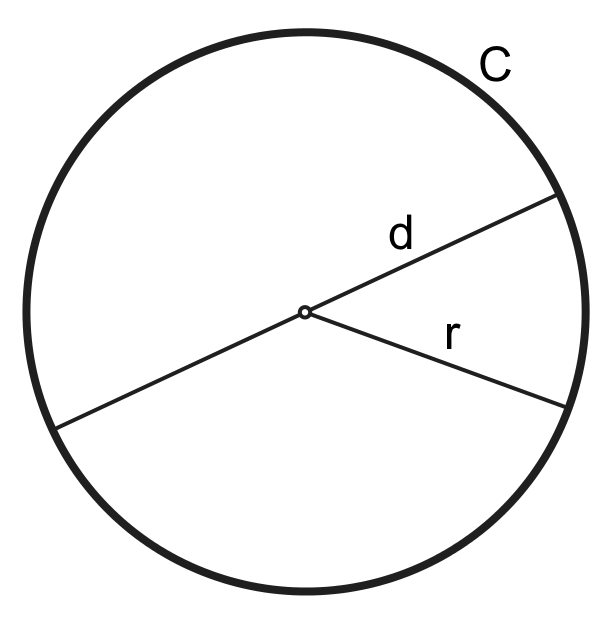# Circle Area Calculator

Use the circle area calculator to calculate the area, diameter, circumference or radius of a circle.

## Circle Area

The length `C` of the circumference (perimeter) of a circle is calculated using the formula:

`C = 2pir`, where `r` is the radius of the circle.

The radius may also be expressed using the diameter so that `2r = d`:

`C=pid`

The area `A` left inside the circle is calculated using the formula:`A=pir^2`, where `r` is the radius of the circle or correspondingly:

`A=pi/4d^2`, where `d` is the diameter of the circle

If the diameter of the circle `d` and the length of the circumference `C` are known, the area can be calculated (without the figure `pi`) using the formula:

`A = Cd/4`

## Information About the Circle Area Calculator

Use the circle area calculator to calculate the area, diameter, circumference or radius of a circle.